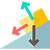top of page### Gravity and Motion

#### Inclined Planes

###### Content ContributorsDev Lohar

# Learning Objectives###### ​###### Normal forceWhen any object exerts a force on a surface, the surface exerts a force on the object and this force is at right angles to the surface. This force is called the reaction force or more commonly known as the normal force. If we consider the weight force exerted by an object on a horizontal surface, then the normal force will be the exact same magnitude as the weight force. But if the surface is on an incline, then the normal force will not be the same as the weight force, and other component forces come into play as explained in the next paragraph.###### Inclined planesAn object on an inclined plane will still slide down the plane, despite you providing any sort of force on it (e.g. pushing it, placing a strong magnet at the bottom of the slope) due to an unbalanced force. This force is a component of the weight force (of the object directed down the slope) and is responsible for the acceleration of the object.

Using the diagram above as a reference (which assumes no friction or other forces), we can establish from prior knowledge that the 2 forces acting upon the object are the weight force acting directly down, and the normal force acting perpendicular to the inclined plane. The weight force can be divided into its 2 perpendicular components. The perpendicular component force acts directly opposite of the normal force and hence balances the normal force, whilst the parallel component is not balanced by any other force, hence becoming the reason which causes the object to accelerate down the incline plane. This parallel component is the net force. Using basic trigonometry, we can calculate the equations for the parallel component and the perpendicular component.###### ​The net force can then be used to calculate the object’s acceleration since we know that F = ma.###### ​If we now include a frictional force or a tension force acting up the slope, then to calculate the net force, all you need to do is take away the frictional force from the parallel component force.###### ​If an object is not accelerating down an inclined plane, then it can be concluded that the frictional force is equal to the parallel component force.###### ​###### ​###### ​bottom of page